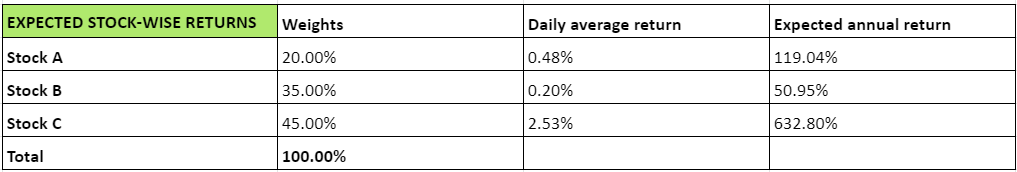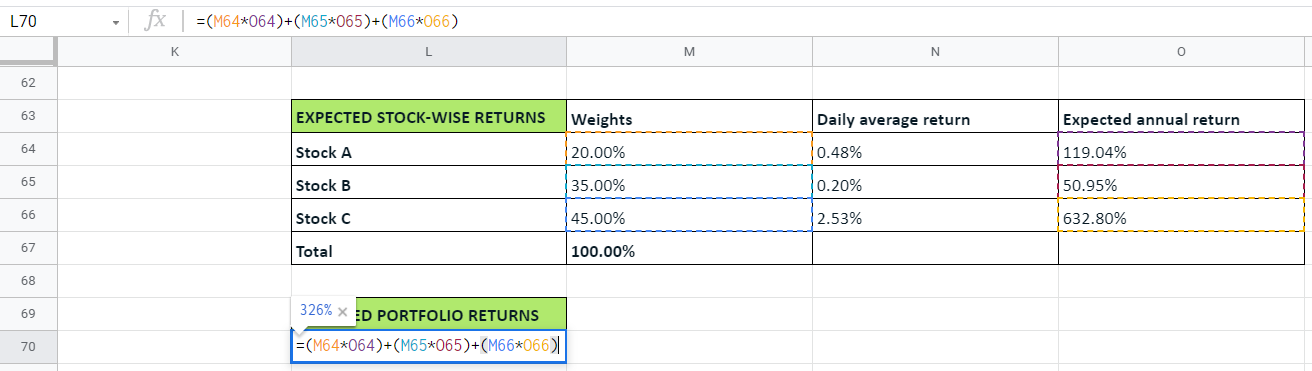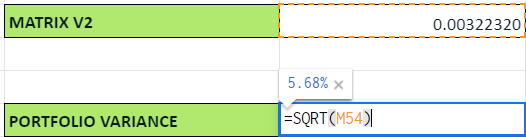Introducing the statistics of risk

Translate the power of knowledge into action. Open Free* Demat Account

# Decoding expected returns and estimating the portfolio range

4.4Up until the previous chapter, we saw the procedure involved in calculating the variance of a portfolio. Now, we’ll discuss the concept of expected returns at a portfolio level. Remember reading about it in the first chapter of this module? Let’s quickly look through a refresher before we proceed.

### What are expected returns?

Simply put, they are the returns that you expect from an investment.

Scenario 1:

• You invest Rs. 200 in stock A, and you expect it to grow to around Rs. 240 after one year.
• So, you essentially expect Rs. 200 to yield a return of Rs. 40.
• The expected return in this case is 20%.

Scenario 2:

• Here, say you have the same R. 200. But instead of investing it all in the same stock, you split your capital.
• You invest Rs. 100 in stock A, and Rs. 100 in stock B.
• You expect stock A to yield a return of 20%, and stock B to yield a return of 10%.
• Basically, you now have a portfolio of two stocks - stock A and stock B.

In this case, what are the expected returns from your portfolio?

You can see that it is neither 20%, nor 10%. Then, how do you figure out the returns from the portfolio?

You can use this formula to calculate it.

 Expected returns from a portfolio = (Weight of asset 1 x Expected returns from that asset) + (Weight of asset 2 x Expected returns from that asset) + ……………. + (Weight of asset n x Expected returns from that asset) +

So, in this case, the expected returns would be:

= (50% x 20%) + (50% x 10%)

= 10% + 5%

= 15%

Now, every portfolio of assets has a range over which it may likely move over the course of the year. As an investor, you need to be able to assess this to a certain degree, so you can plan your investments accordingly. And to do that, we need to begin by calculating the expected returns from the portfolio. Let’s take a quick look at the steps involved before we proceed.

1. Calculate the expected annual return from each stock
2. Compute the expected annual return from the portfolio
3. Calculate the annual portfolio variance
4. Evaluate the portfolio range

### Step 1: Calculate the expected annual return from each stock

Up until now, we’ve calculated the daily average return for each stock. Check out the numbers below, based on the three stocks we’ve been dealing with in our example so far.

• Stock A: 0.48%
• Stock B: 0.20%
• Stock C: 2.53%

This is simply the average of the daily returns we got. Now, in each trading year, the number of trading days is not the same as 365. That’s because the market remains closed duRing weekends and public holidays. In some years, the number of trading days may be 252 or 251, while in others it may be 248 or 249. To make calculations easy, we’ll take 250 as the number of trading days in a year.

So, the expected annual return of each stock will be calculated as follows:

 Expected annual return = The daily average return for each stock x 250

We’ve used excel to calculate this, as you can see in the image below.### Step 2: Compute the expected annual return from the portfolio

Now, you know the expected annual return of each stock. But what about the annual returns from the portfolio as a whole? As you’ll recall from earlier in this chapter, this is the formula you should use.

 Expected returns from a portfolio = (Weight of asset 1 x Expected returns from that asset) + (Weight of asset 2 x Expected returns from that asset) + ……………. + (Weight of asset n x Expected returns from that asset) +

So, when we do that for these 3 stocks we’ve been discussing, here’s what we get as the expected portfolio returns.The expected annual returns from the portfolio is 326%. Keep in mind that we’re working with hypothetical data. So, the question of whether such high returns are possible - we’ll reserve that for another day. Now that we have the annual portfolio returns, we’ll need to compute the annual portfolio variance.

### Step 3: Calculate the annual portfolio variance

Do you recall how we calculated the variance of the portfolio as 5.68%. Here’s a screenshot to rejig your memory.Now, this is the daily portfolio variance, which we obtained by taking the square root matrix V2. To get the annual portfolio variance, let’s first extrapolate matrix V2 from a daily to an annual measure, and then take its square root.

 Particulars Calculation Value Matrix V2 (daily) 0.00322320 Matrix V2 (annual) = Daily V2 * 250 trading days = 0.00322320 x 250 0.80579906 Annual portfolio variance = Square root of annual V2 = Square root of 0.80579906 89.77%

So, the annual portfolio variance in this case = 89.77%.

### Step 4: Evaluate the portfolio range

Once again, we’ll revisit the normal distribution and the empirical rule to estimate the range of returns you can expect from this portfolio. There are the two key data points.

• Expected annual returns from the portfolio = 326%.
• Annual portfolio variance = 89.77%.

And the empirical rule states that:

• 68% of the time, the returns will vary by 1SD on either side.
• 95% of the time, the returns will vary by 2SD on either side.
• 99% of the time, the returns will vary by 3SD on either side.

Here, the annual portfolio variance of 89.77% corresponds to 1SD. Let’s extrapolate and find what 2D and 3SD will be.

 Portfolio variance at 1 SD Portfolio variance at 2 SD Portfolio variance at 3 SD 89.77% 179.53% 269.30%

Now, coming to the range of returns you can expect from this portfolio, we’ll need an upper limit and a lower limit for any range, right? Based on normal distribution, this is how the range of expected returns looks at different degrees of certainty.

 Particulars Lower limit of returns Upper limit of returns Portfolio range of expected returns Case 1:68% of the time Expected annual return - 1 SD = 326% - 89.77% = 236% Expected annual return + 1 SD = 326% + 89.77% = 416% 236% to 416% Case 2:95% of the time Expected annual return - 2 SD = 326% - 179.53% = 146% Expected annual return + 2 SD = 326% + 179.53% = 506% 146% to 506% Case 3:99% of the time Expected annual return - 3 SD = 326% - 269.30% = 57% Expected annual return + 3 SD = 326% + 269.30% = 596% 57% to 596%

#### Wrapping up

This is how you can estimate the expected annual returns from your portfolio with varying levels of certainty. Bear in mind that this is not an absolute set of data. It is just a set of estimates that can help you make investment decisions in a more informed manner. Also, here’s an interesting thing to note. The more the probability, the wider the range. So, if you want to narrow the expected returns to a smaller range, the level of certainty comes down. This means the level of risk goes up.

#### A quick recap

• The overall returns of the portfolio depend on the weights of the individual stocks and their average annual returns.
• You can arrive at the annual expected portfolio returns by taking the sum of the products of individual stock’s returns and its weight.
• The daily variance of the portfolio can be extrapolated to the annual variance by multiplying it with the square root of 25o.
• Then, using the normal distribution pattern, you can arrive at 1SD, 2SD and 3D.
• To get the lower limit of the range of expected returns, you need to subtract the variance from the portfolio’s expected return.
• To get the upper limit of the range of expected returns, you need to add the variance to the portfolio’s expected return.
• Then, using the empirical rule, you can find the range with varying levels of certainty.